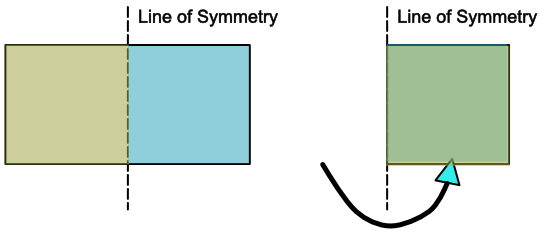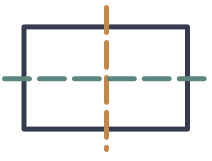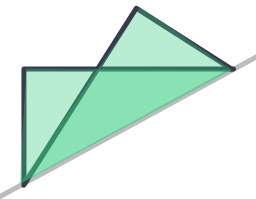Polygons with Symmetries

# Polygons with Symmetries

GCSE(F),

Polygons may have symmetry. Symmetry means that things are equal, or balanced. In Geometry, there are two types of symmetry: Lines of Symmetry and Rotational Symmetry.A polygon may have one or more lines of symmetry. If the polygon was folded along a line of symmetry, then each half of the polygon would match exactly.A polygon may also have rotational symmetry. If the polygon is rotated about a point and it then looks like itself after being rotated, then it has rotational symmetry. The number of positions where this happens gives the Order of Rotational Symmetry: if it can be rotated three times to look like itself, then it has an Order of Rotational Symmetry of value 3.

A polygon with an order of rotational symmetry equal to 1 does not have rotational symmetry.

## Examples

1. How many lines of symmetry does a rectangle have?A rectangle has two lines of symmetry (it can be folded on the line of symmetry to match the other side).

2. A line is drawn across a rectangle as shown below. Is it a line of symmetry?